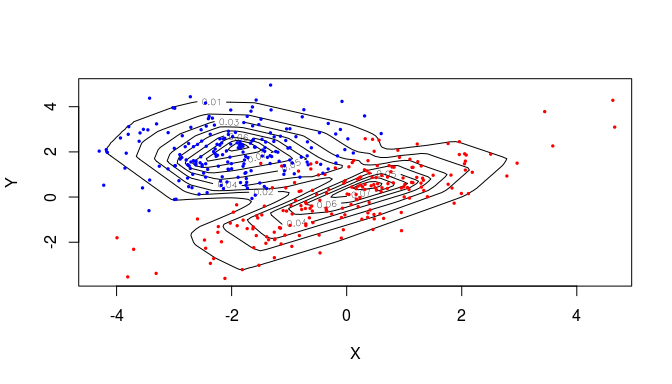# Usage of fmlogcondens

#### 2018-03-23

This document introducess the usage of the package fmlogcondens. The package name stands for (f)ast (m)ultivariate (log)-(con)cave (dens)ity estimation. It estimates a non-pararametric density, whose logarithm is a concave function. The class of log-concave probability distributions includes many well known parametric densities such as normal distributions, exponential distributions, Wishart distributions, Gamma distributions with shape parameter larger than one and many more (Walther and others 2009).

The second part of this document introduces all relevant functions of the package for obtaining and plotting estimates of log-concave and mixtures of log-concave densities. The first part provides some theoretical background of the maximum likelihood estimator and how it is approached algorithmically. It also compares this package to two relevant R packages: logcondens (Dümbgen, Hüsler, and Rufibach 2007) that can be used for univariate data and LogConcDEAD (Cule, Samworth, and Stewart 2010) which adresses the multivariate case, though has significanlty longer runtimes. A detailed numerical comparison can be found in our publication (Rathke and Schnörr 2015).

## Background information

The estimation of a log-concave density can be formulated as the maximum likelihood problem for any sample $$X$$ consisting of $$n$$ points $$X_i \in \mathbb{R}^d$$.

$\hat{f}_n = \text{argmax}_{f} \; \sigma(f) \qquad \sigma(f) = \frac{1}{n} \sum_{i=1}^n \log f(X_i) + \int f(x) dx$ The integral term is a Lagrange term that guarantees that $$\hat{f}_n$$ is a normalized density. Finding the MLE $$\hat{f}_n$$ is significantly simplified by the fact, that solutons $$\log{\hat{f}_n}$$ take the form of piecwise-linear functions. Furthermore, (Cule, Samworth, and Stewart 2010) went on to show that $$\hat{f}_n$$ is uniquely determined by $$X$$ and a vector $$y \in \mathbb{R}^n$$, where $$y_i = \log f(X_i)$$. Consequently, they formulate the MLE problem in terms of $$y$$:

$\sigma(y) = \frac{1}{n} \sum_{i=1}^n y_i + \int f_y(x) dx, \qquad \log f_y(x) = \sup_{\lambda}\left\{\sum_{i=1}^n \lambda_iy_i \;\Big|\; x = \sum_{i=1}^n \lambda_i X_i, \sum_{i=1}^n \lambda_i = 1, \lambda_i \geq 0 \right\}.$ Their package LogConcDEAD implements a solver for this objective function. The major drawback of their approach is a long running time for large samples ($$\geq$$ 250 points). For example for 10000 samples in 2-D their approach runs for hours while our approach is done in a matter of seconds.

A piecewise concave linear function can be equivalently defined in terms of its slopes $$a$$ and intercepts $$\beta$$. We propose the reformulation of the MLE in terms of these hyperplane parameters:

$\sigma(a,\beta) = \frac{1}{n} \sum_{i=1}^n \log f_{a,\beta}(X_i) + \int f_{a,\beta} (x) dx, \qquad \log f_{a, \beta} (x) = \min_k (a_k^Tx - \beta_k)$ This has two significant advantages: We demonstrate in (Rathke and Schnörr 2015) how this leads to sparse representations (in terms of the number of hyperplanes), which speeds up calculations significantly. Also our objective is smooth, which allows us to use a quasi-Newton optimization method adapted to large scale optimization problems (BFGS-L) (Nocedal and Wright 2006). While our formulation is non-convex contrary to the one of Cule et al., we proove numerically that our approach yields solutions that are very close to the optimum in much less time.

### Univariate log-concave density

For samples in one dimension, the R package logcondens analytically yields the optimal density with the minimal number of hyperplanes.

## Installation

### Standard installation

The most convenient way to install the package is:

install.packages("fmlogcondens")

The drawback of this approach is that the installed binaries where compiled for generic processors, which exclude AVX optimizations. See the next section, on how to activate AVX (and speedup the code by a factor of ~5x).

### Installation with AVX support (Linux and Mac OS only)

AVX is the successor to SSE and MMX, and denotes the ability of modern CPUs to do certain simple computations (addition, multiplication, …) much faster due to specialized instructions. To activate AVX, we have to install the package from source. To do this, we first download the source code from CRAN: link (Package source: fmlogondens_x.x.x.tar.gz).

Then in your favorite text editor open the file ~/.R/Makevars (create it if it does not exist) and add the line

CFLAGS += -march=native

This activates processor specific optimizations for the compilation of C code. [NOTE: This flag will affect all future installations that are manually compiled from source. In general it is a good idea to keep this flag after installing this pacakge, since it may potentially lead to faster code for other future packages too.]

Finally, from within R, we run the command:

install.packages("path-to-package/fmlogcondens_1.0.0.tar.gz", type = "source")

Alternatively we can execute the following command from the shell

R CMD INSTALL path-to-package/fmlogcondens_1.0.0.tar.gz

You can check whether AVX was activated for the installation by calling the function compilationInfo() in R after loading the package. The desired output would be AVX vector extensions activated..

### A note on OpenMP and MacOS

OpenMP (Open Multi-Processing) is a API for C that supports multi-threaded programming. fmlogcondens automatically supports OpenMP. Problems can arise for MacOS when compiling from source, since the clang compiler may not support OpenMP. In that case you can try to follow this tutorial for a guide how to setup OpenMP under MacOS.

## Usage

### Estimating a log-concave density

Load the package in R with:

library(fmlogcondens)

We start with a small example of 100 points in 2-D. We sample from a normal density with zero mean and identity covariance matrix.

set.seed(222)
X = matrix(rnorm(200), 100, 2)
r <- fmlcd(X) # estimate log-concave density

The logarithm of the density f(x) is a piecewise-linear concave function and is parametrized by a set of hyperplane parameters (see Background). r$a contains the normal vectors of all hyperplanes whereas r$b containts their intercepts. r$logMLE holds the log-likelihoods for all data points X_i. Additionally, a sparse representation is returned in r$aSparse and r$bSparse. This corresponds to the final solution of the Newton optimization. In a post-processing step we analytically normalize this sparse representation, thereby losing the sparseness property. Thus while only numerically normalized on the interation grid, the sparse representation can be usefull for qualitative insights into the estimated density. ### Plot the estimated density We can plot the resulting density using functionality of the package LogConcDEAD. In order to be able to use all functions in their package, we have to create an object of class LogConvDEAD using the function getinfolcd(). We can then use the function plot() to display the estimated density as well as the log-density. library(LogConcDEAD) r <- LogConcDEAD::getinfolcd(X, r$logMLE) # create a LogConcDEAD object

par(mfrow = c(1, 2)) #square plots
plot(r, addp = FALSE, asp = 1, main="density")
plot(r, uselog = TRUE, addp = FALSE, asp = 1, main="log density")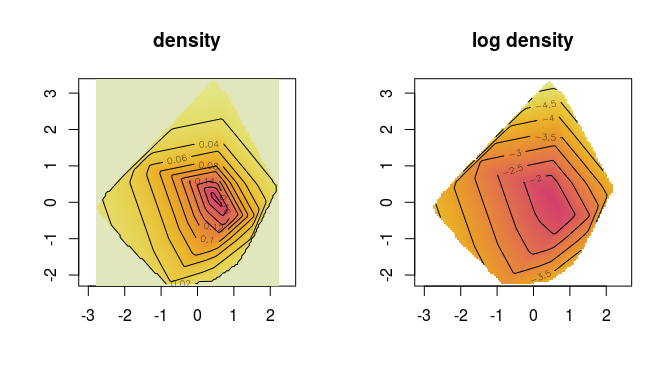### Evaluate the estimated density

Other useful functions are provided by the package LogConcDEAD: To evaluate the estimated density $$f(x)$$ at a point $$x$$, we use the function LogConcDEAD::dlcd. To draw a sample from $$f(x)$$, use LogConcDEAD::rlcd.

# evaluate density for the point (0,0)
x <- c(0,0)
LogConcDEAD::dlcd(x,r)
#>  0.1425058
# sample 10 points from the estimated density
LogConcDEAD::rlcd(10,r)
#>             [,1]        [,2]
#>  [1,]  0.3500454 -0.22966601
#>  [2,] -0.5817292 -1.67077236
#>  [3,]  0.8646483 -0.09962265
#>  [4,] -0.3106089  1.04478556
#>  [5,] -1.7869274  0.82270313
#>  [6,]  1.0773911 -0.16541720
#>  [7,] -0.2298689  0.90146602
#>  [8,]  0.6633195 -1.20953739
#>  [9,]  1.2487350  0.22067672
#> [10,] -2.1438764  0.15982323

### Comparing with the density estimate from LogConcDEAD

We now estimate the log-concave density $$\hat{f}_n$$ with maximal log likelihood using the package LogConcDEAD. Remember that their approach yields the optimal solution for the MLE problem. Comparing this estimate with the one from our package reveals that our optimization approach yielded near optimal results.

rCule <- LogConcDEAD::mlelcd(X)

par(mfrow = c(1, 2)) #square plots
plot(rCule, addp=  FALSE, asp = 1, main = "density [LogConcDEAD]")
plot(rCule, uselog = TRUE, addp = FALSE, asp = 1, main = "log density [LogConcDEAD]")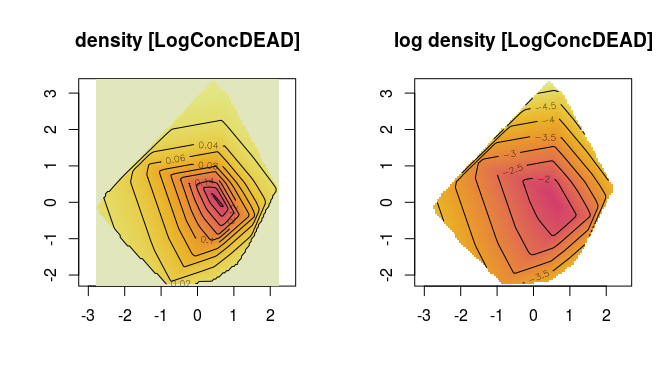### Comparing the performance for larger samples

We will now increase the sample size, in order to demonstrate the advantages of our packages: the very fast computation of $$\hat{f}_n$$. To this end, we estimate the log-concave density for 500 data points in two dimensions using using both approaches.

set.seed(222)
X = matrix(rnorm(1000), 500, 2)
# time estimate for our approach
system.time(r <- fmlcd(X))
#>    user  system elapsed
#>   1.448   0.032   0.512
# time estimate for the approach of Cule et al.
system.time(rCule <- mlelcd(X))
#>    user  system elapsed
#>  20.348   0.336  20.783

We see how the required runtime differs in a magnitude of more than ten (less without AVX enabled, see AVX). Run this example for larger sample sizes to see the ratio grow beyond ten thousand.

We now plot the estimated densities and see that they are almost identical:

r <- LogConcDEAD::getinfolcd(X, r$logMLE) # create a LogConcDEAD object # plot bost estimates for comparison par(mfrow=c(1, 2)) #square plots plot(r, addp = FALSE, asp = 1, main="density") plot(rCule, addp = FALSE, asp = 1, main="density [LogConcDEAD]")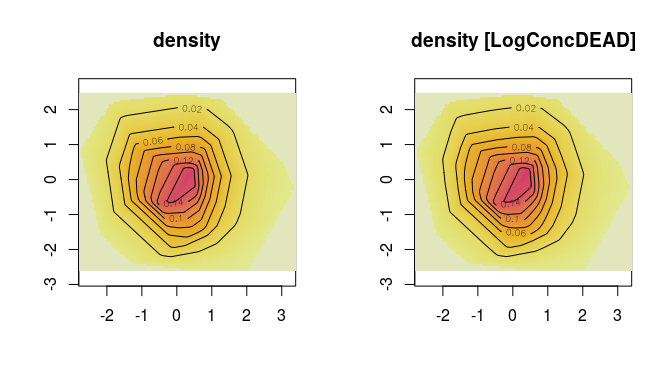### Estimating a mixture of log-concave densities Finally, we demonstrate the ability of to estimate mixtures of log-concave densities. To this end, we sample data points from two normal densities with different parameters. library(MASS) set.seed(222) X1 <- mvrnorm(200, c(0, 0), matrix(c(2, 1.5, 1.5, 2), 2, 2)) X2 <- mvrnorm(200, c(-2, 2), matrix(c(1, 0, 0, 1), 2, 2)) plot(X1[ ,1], X1[ ,2], col="red", pch = 20, xlab = "X", ylab = "Y") points(X2[ ,1], X2[ ,2], col="blue", pch = 20)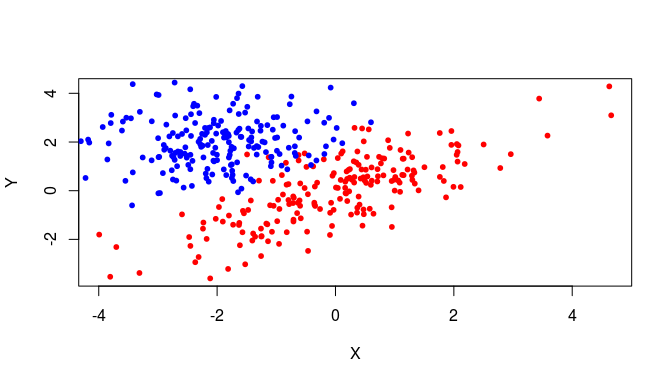Estimate a mixture density having K=2 classes. X <- rbind(X1,X2) # stack both data matrices r <- fmlcdEM(X, K = 2) Finally, we evaluate the mixture density for a grid of points and plot the results. # Create a grid of points for evaluation x <- seq(min(X[ ,1]), max(X[ ,1]), 0.1) y <- seq(min(X[ ,2]), max(X[ ,2]), 0.1) m <- length(x); n <- length(y) XEval = cbind(matrix(rep(x, each = n), ncol = 1), matrix(rep(y, m), ncol = 1)) nX = dim(XEval) # evaluate log-concave density component-wise YA = exp(apply(- r$params[]$a %*% t(XEval) - matrix(rep(r$params[]$b,nX),length(r$params[]$b),nX), 2, min)) YB = exp(apply(- r$params[]$a %*% t(XEval) - matrix(rep(r$params[]$b,nX),length(r$params[]$b),nX), 2, min)) Y = YA * r$tau + YB * r\$tau

contour(x,y,t(matrix(Y,n,m)), xlab = "X", ylab = "Y")
points(X1[ ,1], X1[ ,2], col="red", pch = 20, cex=.5)
points(X2[ ,1], X2[ ,2], col="blue", pch = 20, cex=.5)